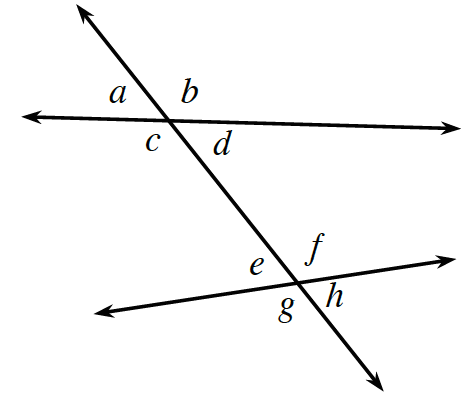### Home > INT2 > Chapter 1 > Lesson 1.3.3 > Problem1-98

1-98.

Using the diagram at right, name the angle pair relationships of the angle pairs listed below.

angle possibilities: supplementary, vertical, corresponding, straight pair, alternate interior

1. $d$ and $e$

1. $e$ and $h$

1. $a$ and $e$

1. $c$ and $d$